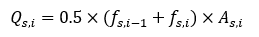top of page

# Methodology for Axial Capacity Calculation in PileAXL Program

Axial capacity of piles (Q) in the PileAXL program consists of shaft resistance (Qs) along the pile shaft and end bearing resistance (Qb) below the pile toe as per the equation below:The figure below shows the shaft resistance and end bearing resistance for axial capacity calculation of the closed-ended piles.For open-ended piles, both plugged and unplugged axial capacity can be calculated by the program. The following figure shows the shaft resistance and end bearing resistance for axial capacity calculation of the open-ended piles for plugged conditions.Plugged axial capacity (Q-plugged) is the sum of external shaft resistance (Q-s,o) and end bearing resistance (Q-b,f) based on the full section area with the equation below:Unplugged axial capacity (Q-unplugged) is the sum of external (Q-s,o) and internal (Q-s,i) shaft resistance and end bearing resistance (Q-b,a) based on the annual section area.where Beta is a reduction factor for the internal shaft resistance (Q-s,i) and can be defined by the users in the program. Di is the internal diameter and Do is the external diameter. The following figure shows the shaft resistance and end bearing resistance for axial capacity calculation of the open-ended piles for unplugged conditions.For the piles subject to tensile load at pile head, the axial tension capacity is shaft resistance only in the PileAXL program and is determined with the equation below:In PileAXL program, the maximum input pile length is divided equally into NE elements for calculating the axial capacity of different pile lengths. The following figure shows the nodes and elements along the maximum pile length.At each node position, the program will calculate the ultimate unit shaft resistance (fsi) and ultimate unit end bearing resistance (fbi).

For each pile element, the ultimate shaft resistance force (Q-s,i) is estimated with the following equation:where A-s,i is the shaft area of the pile element.

At the bottom of each pile element, the ultimate end bearing resistance (Q-b,i) is estimated with the following equation:where A-b,i is the pile toe area of the pile element, .

The program will calculate the axial capacity for (NE+1) numbers of pile lengths based on the input maximum pile length (Lmax) and element number (NE). The axial capacity (Qi) for a specific pile length (Li) is determined with the following equation:where i starts from 1 to NE+1.

143 views